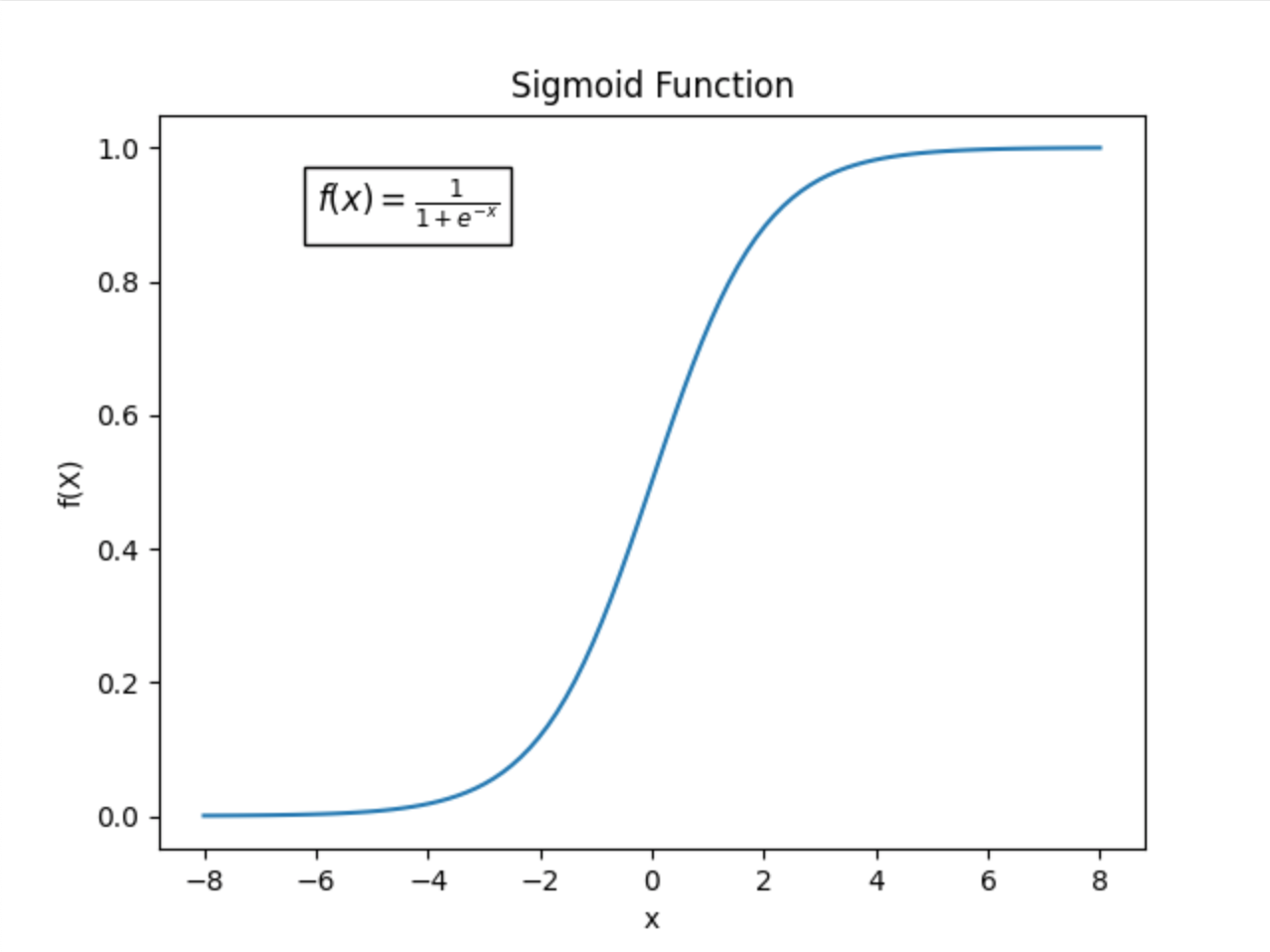# Sigmoid Activation Function

A sigmoid activation function is a specific type of sigmoid function commonly used in machine learning and various fields of artificial intelligence (AI). They play a crucial role in machine learning by enabling the development of neural networks for many machine learning tasks. In other areas of AI, sigmoid activation functions can be applied to probabilistic modeling, decision making, uncertainty modeling, and more.

## Sigmoid Functions

A sigmoid function is a type of mathematical function that is commonly used in AI, specifically in machine learning. The graph of a sigmoid function is characterized by its S-shaped curve or “sigmoid curve”.Sigmoid functions transform data by mapping input values to a range between 0 and 1. The output of a sigmoid function can be interpreted as a value that represents the probability or intensity of an event. This makes sigmoid functions useful for representing relationships in data, as they can provide a measure of how likely or strong something is based on the input.

## Sigmoid Activation Functions in Machine Learning

A sigmoid activation function refers to the use of a sigmoid function as an activation function in neural networks. Activation functions are like decision-making tools used in machine learning. They help determine if specific neurons in a neural network should be activated or not based on the input they receive.

Sigmoid activation functions are commonly used in machine learning because they help neural networks understand and learn from complex patterns in data. They introduce non-linearity to the data, which means the relationship between input and output is not a simple straight line. Instead, sigmoid activation functions can help neural networks capture intricate and non-linear relationships. This is important because problems that are encountered in the real-world, such as image recognition or language processing, often have behaviors that are not straightforward or linear. Sigmoid activation functions allow the neural network to handle these complexities.

## Practical Uses for Sigmoid Activation Functions

Sigmoid activation functions can be used for many practical machine learning tasks, such as:

• Binary classification: Sigmoid activation functions are especially useful in binary classification tasks, where samples are assigned to one of two classes. In such tasks, a threshold (typically 0.5) can be set so that values above the threshold are classified into one class, and values below the threshold are classified into the other class.
• Sentiment analysis: Sentiment analysis aims to determine the emotion, or sentiment, of text data. Sigmoid activation functions can be used to assign probabilities to different sentiment classes, such as positive or negative sentiment.
• Fraud detection: Sigmoid activation functions can be used in neural networks to detect the likelihood of a transaction being fraudulent based on multiple transaction features.
• Financial risk assessment: Sigmoid activation functions can be used in neural networks to assign probabilities to different risk factors, such as credit risk or the likelihood of loan defaults.